# 吴恩达《Machine Learning》Jupyter Notebook 版笔记发布！

https://www.coursera.org/learn/machine-learning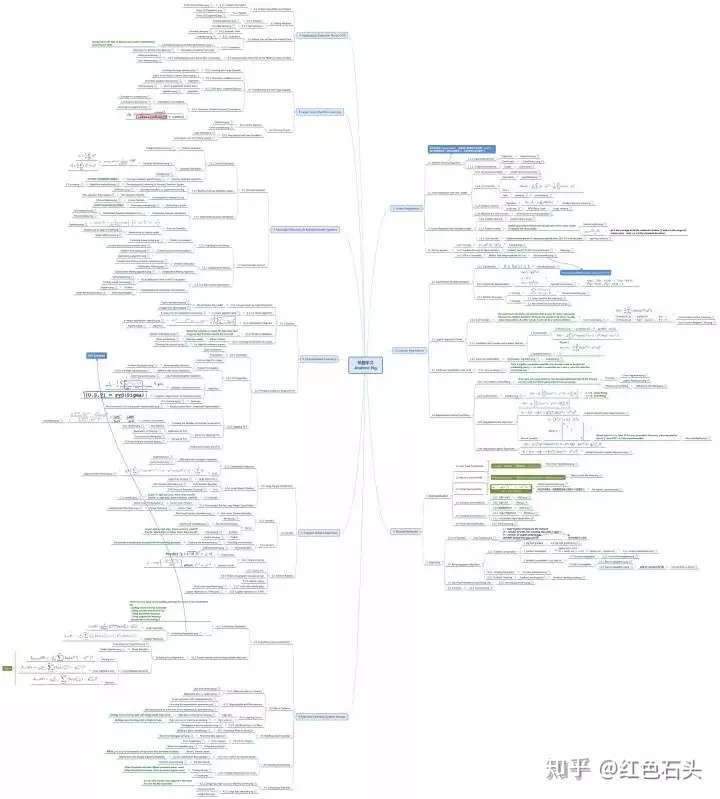https://pan.baidu.com/s/16065BpNAP7JEx_PpFHLSOw

https://github.com/halfrost/Halfrost-Field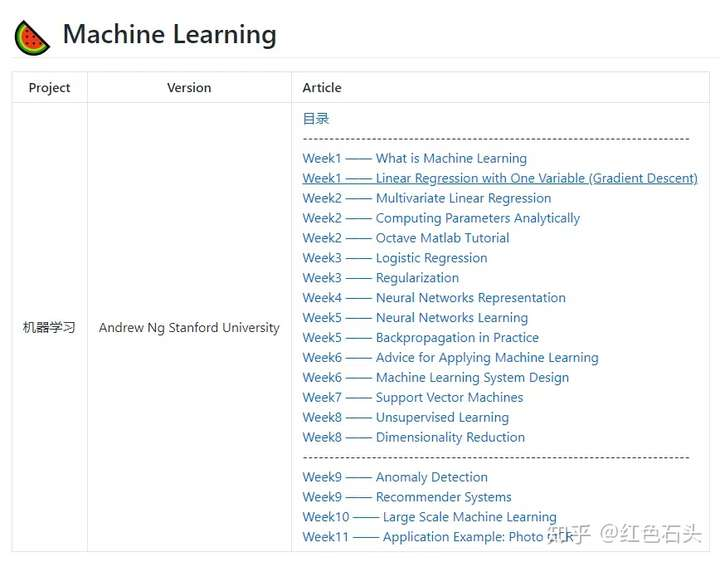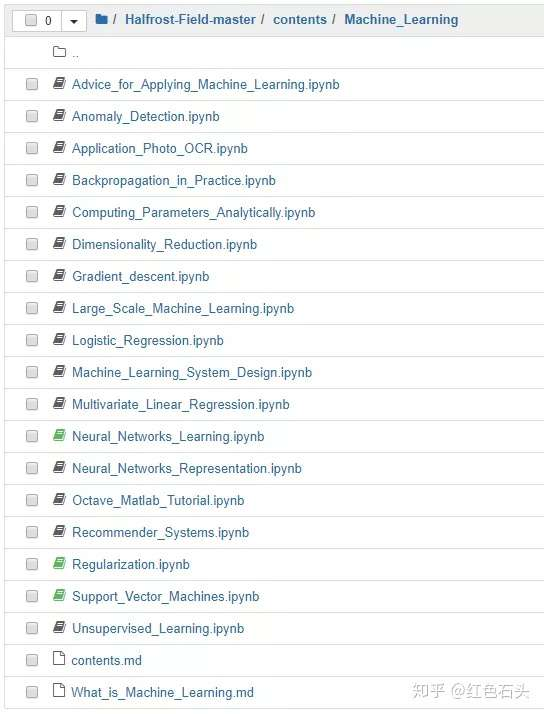## 1. 支持向量机（SVM）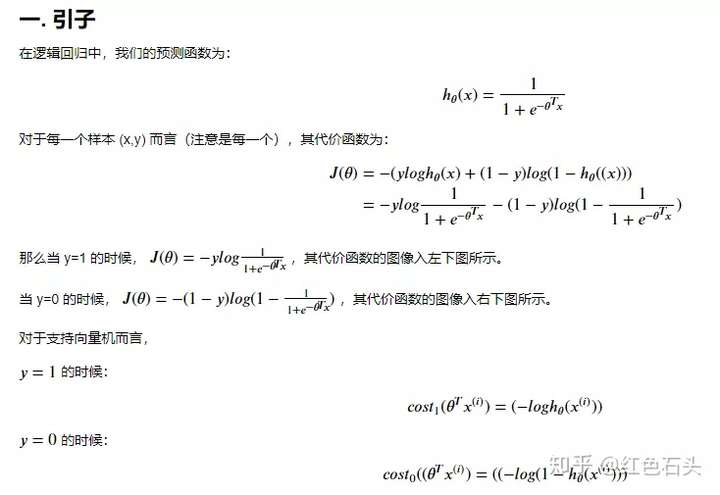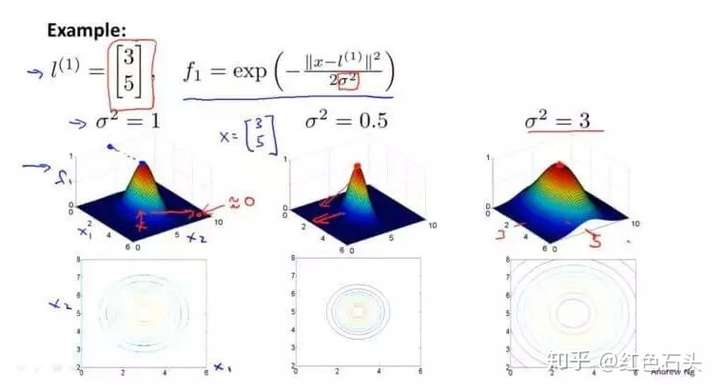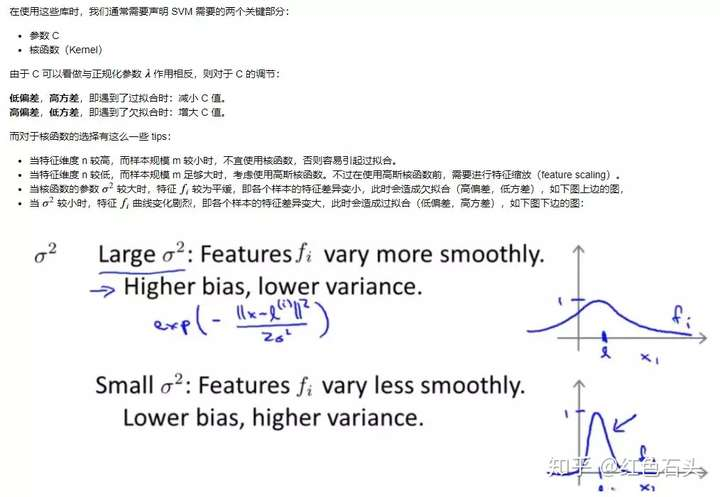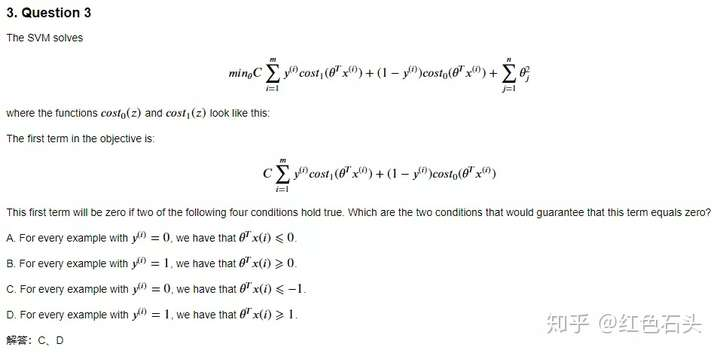## 2. 神经网络学习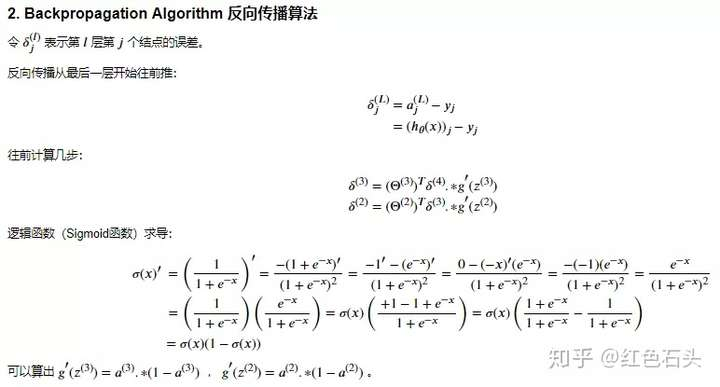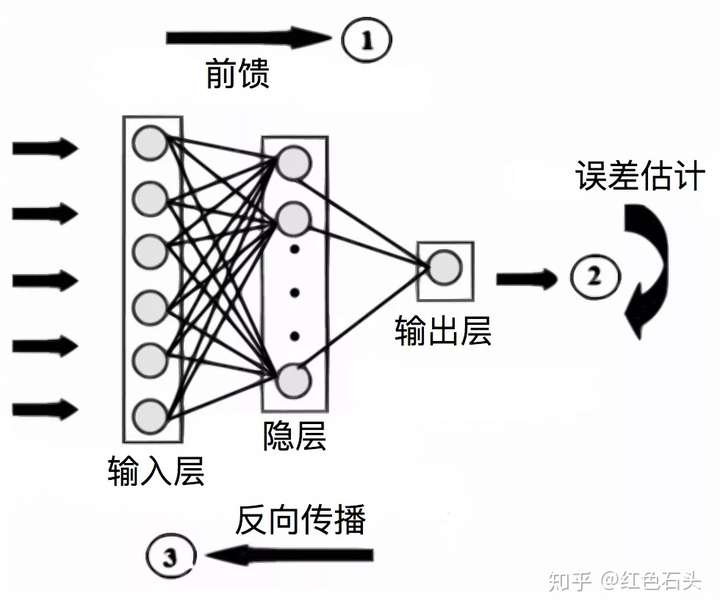function g = sigmoid(z)
g = 1.0 ./ (1.0 + exp(-z));
end

g = sigmoid(z) .* (1 - sigmoid(z));
end

delta3 = a3 - Y;

Theta2_grad = 1 / m * delta3' * [ones(m, 1), a2] + ...
lambda / m * [zeros(K, 1), Theta2(:, 2:end)]; # 正规化部分

delta2 = (delta3 * Theta2 .* sigmoidGradient([ones(m, 1), z2]));
delta2 = delta2(:, 2:end); # 反向计算多一个偏移参数误差，除去

Theta1_grad = 1 / m * delta2' * [ones(m, 1), a1] + ...
lambda / m * [zeros(H, 1), Theta1(:, 2:end)]; # 正规化部分


## 附加资源：

https://github.com/nsoojin/coursera-ml-py

https://github.com/kaleko/CourseraML

1. 扫描下方二维码并关注

2. 公众号后台回复关键词：NGjupyter### 觉得文章有用就打赏一下文章作者

#### 支付宝扫一扫打赏#### 微信扫一扫打赏